# tidyterraThe goal of {tidyterra} is to provide common methods of the tidyverse packages for objects created with the {terra} package: SpatRaster and SpatVector. It also provides geoms for plotting these objects with {ggplot2}.

## Overview

{tidyverse} methods implemented on {tidyterra} works differently depending on the type of Spat* object:

• SpatVector: the methods are implemented using `terra::as.data.frame()` coercion. Rows correspond to geometries and columns correspond to attributes of the geometry.

• SpatRaster: The implementation on SpatRaster objects differs, since the methods could be applied to layers or to cells. {tidyterra} overall approach is to treat the layers as columns of a tibble and the cells as rows (i.e. `select(SpatRaster, 1)` would select the first layer of a SpatRaster).

The methods implemented return the same type of object used as input, unless the expected behavior of the method is to return another type of object, (for example, `as_tibble()` would return a tibble).

Current methods and functions provided by {tidyterra} are:

tidyverse method SpatVector SpatRaster
`tibble::as_tibble()` ✔️ ✔️
`dplyr::select()` ✔️ ✔️ Select layers
`dplyr::mutate()` ✔️ ✔️ Create /modify layers
`dplyr::transmute()` ✔️ ✔️
`dplyr::filter()` ✔️ ✔️ Modify cells values and (additionally) remove outer cells.
`dplyr::slice()` ✔️ ✔️ Additional methods for slicing by row and column.
`dplyr::pull()` ✔️ ✔️
`dplyr::rename()` ✔️ ✔️
`dplyr::relocate()` ✔️ ✔️
`dplyr::distinct()` ✔️
`dplyr::arrange()` ✔️
`dplyr::glimpse()` ✔️ ✔️
`dplyr::inner_join()` family ✔️
`dplyr::summarise()` ✔️
`dplyr::group_by()` family ✔️
`dplyr::rowwise()` ✔️
`dplyr::count()`, `tally()` ✔️
`dplyr::bind_cols()` / `dplyr::bind_rows()` ✔️ as `bind_spat_cols()` / `bind_spat_rows()`
`tidyr::drop_na()` ✔️ ✔️ Remove cell values with `NA` on any layer. Additionally, outer cells with `NA` are removed.
`tidyr::replace_na()` ✔️ ✔️
`ggplot2::autoplot()` ✔️ ✔️
`ggplot2::fortify()` ✔️ to sf via `sf::st_as_sf()` To a tibble with coordinates.
`ggplot2::geom_*()` ✔️ `geom_spatvector()` ✔️ `geom_spatraster()` and `geom_spatraster_rgb()`.

## :exclamation: A note on performance

{tidyterra} is conceived as a user-friendly wrapper of {terra} using the {tidyverse} methods and verbs. This approach therefore has a cost in terms of performance.

If you are a heavy user of {terra} or you need to work with big raster files, {terra} is much more focused on terms of performance. When possible, each function of {tidyterra} references to its equivalent on {terra}.

As a rule of thumb if your raster has less than 10.000.000 data slots counting cells and layers (i.e. `terra::ncell(your_rast)*terra::nlyr(your_rast) < 10e6`) you are good to go with {tidyterra}.

When plotting rasters, resampling is performed automatically (as `terra::plot()` does, see the help page). You can adjust this with the `maxcell` parameter.

## Installation

Install {tidyterra} from CRAN:

``install.packages("tidyterra")``

You can install the development version of {tidyterra} like so:

``remotes::install_github("dieghernan/tidyterra")``

Alternatively, you can install {tidyterra} using the r-universe:

``````# Enable this universe
options(repos = c(
dieghernan = "https://dieghernan.r-universe.dev",
CRAN = "https://cloud.r-project.org"
))
install.packages("tidyterra")``````

## Example

### SpatRasters

This is a basic example which shows you how to manipulate and plot SpatRaster objects:

``````library(tidyterra)

library(terra)

# Temperatures
f <- system.file("extdata/cyl_temp.tif", package = "tidyterra")

rastertemp <- rast(f)

library(ggplot2)

# Facet all layers

ggplot() +
geom_spatraster(data = rastertemp) +
facet_wrap(~lyr, ncol = 2) +
scale_fill_whitebox_c(
palette = "muted",
labels = scales::label_number(suffix = "º")
) +
labs(fill = "Avg temperature")``````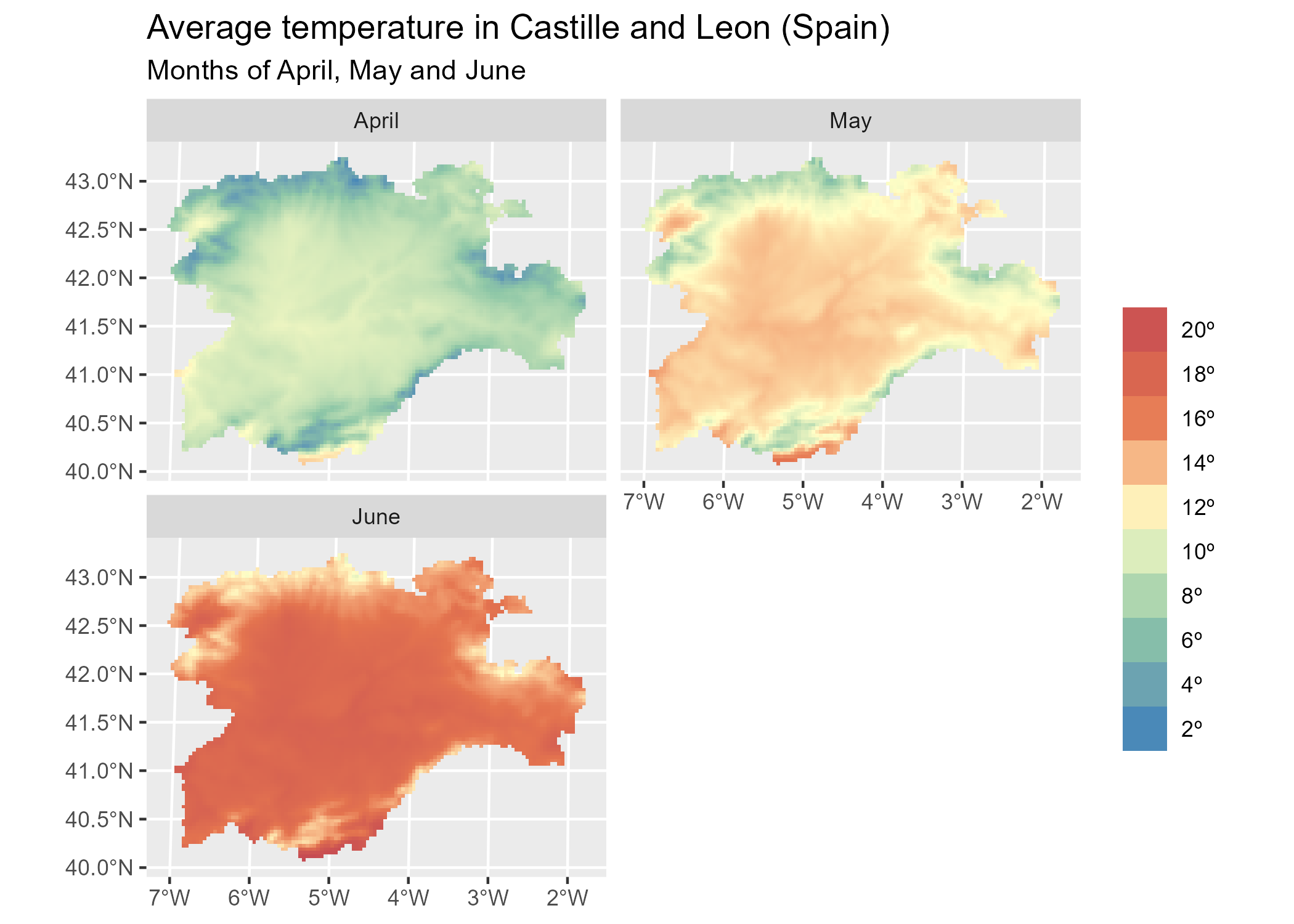``````

# Create maximum differences

variation <- rastertemp %>%
mutate(
diff = tavg_06 - tavg_04
) %>%
select(var_apr_jun = diff)

# Add also a overlay of a SpatVector
f_vect <- system.file("extdata/cyl.gpkg", package = "tidyterra")

prov <- vect(f_vect)

ggplot(prov) +
geom_spatraster(data = variation) +
geom_spatvector(fill = NA) +
scale_fill_whitebox_c(
palette = "deep", direction = -1,
labels = scales::label_number(suffix = "º")
) +
theme_minimal() +
coord_sf(crs = 25830) +
labs(
fill = "Difference",
title = "Variation of temperature in Castille and Leon (Spain)",
subtitle = "(Average) temperatures in June vs. April"
)``````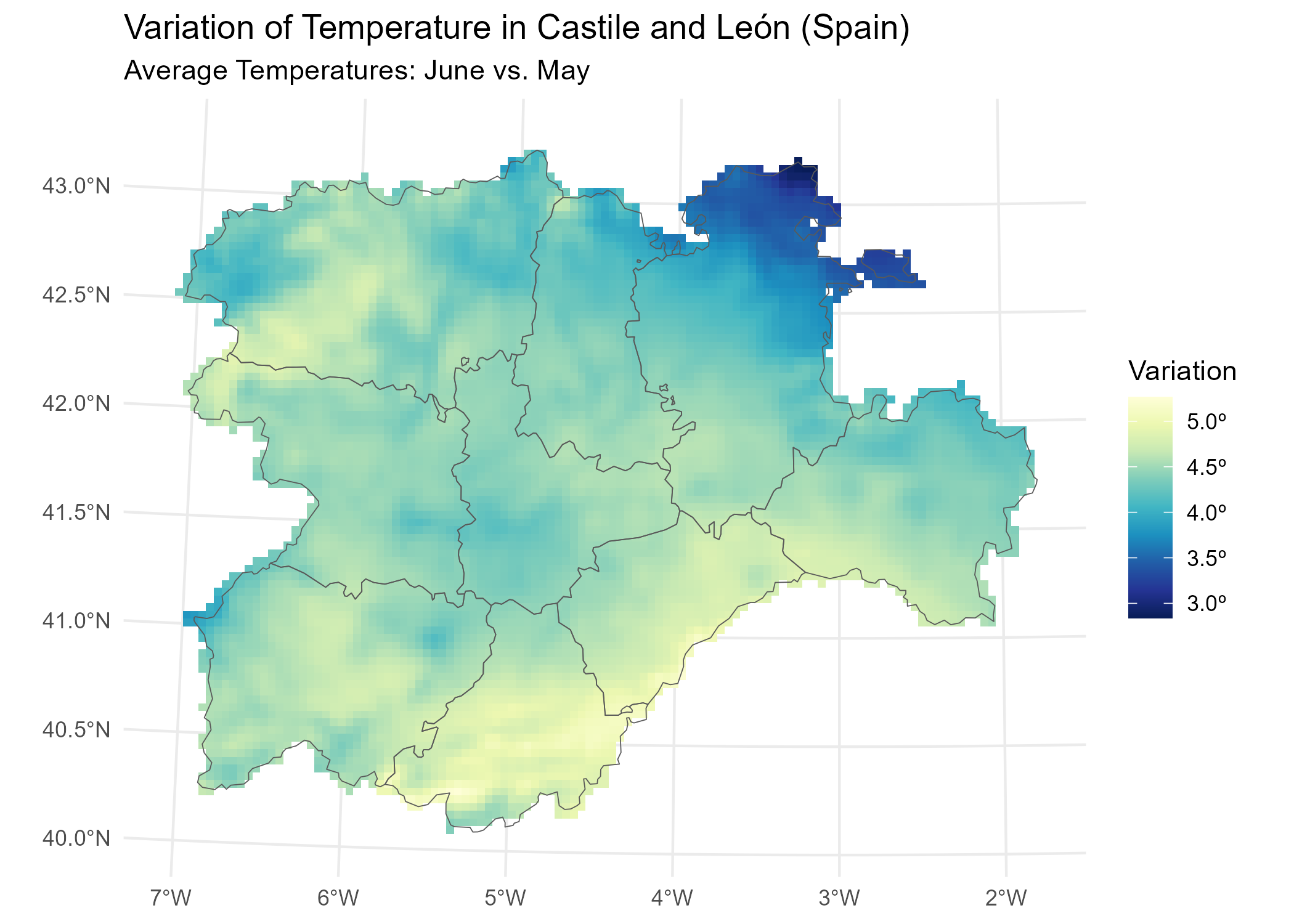{tidyterra} also provide a geom for plotting RGB SpatRaster tiles with {ggplot2}

``````f_tile <- system.file("extdata/cyl_tile.tif", package = "tidyterra")

rgb_tile <- rast(f_tile)

plot <- ggplot(prov) +
geom_spatraster_rgb(data = rgb_tile) +
geom_spatvector(fill = NA) +
theme_light()

plot``````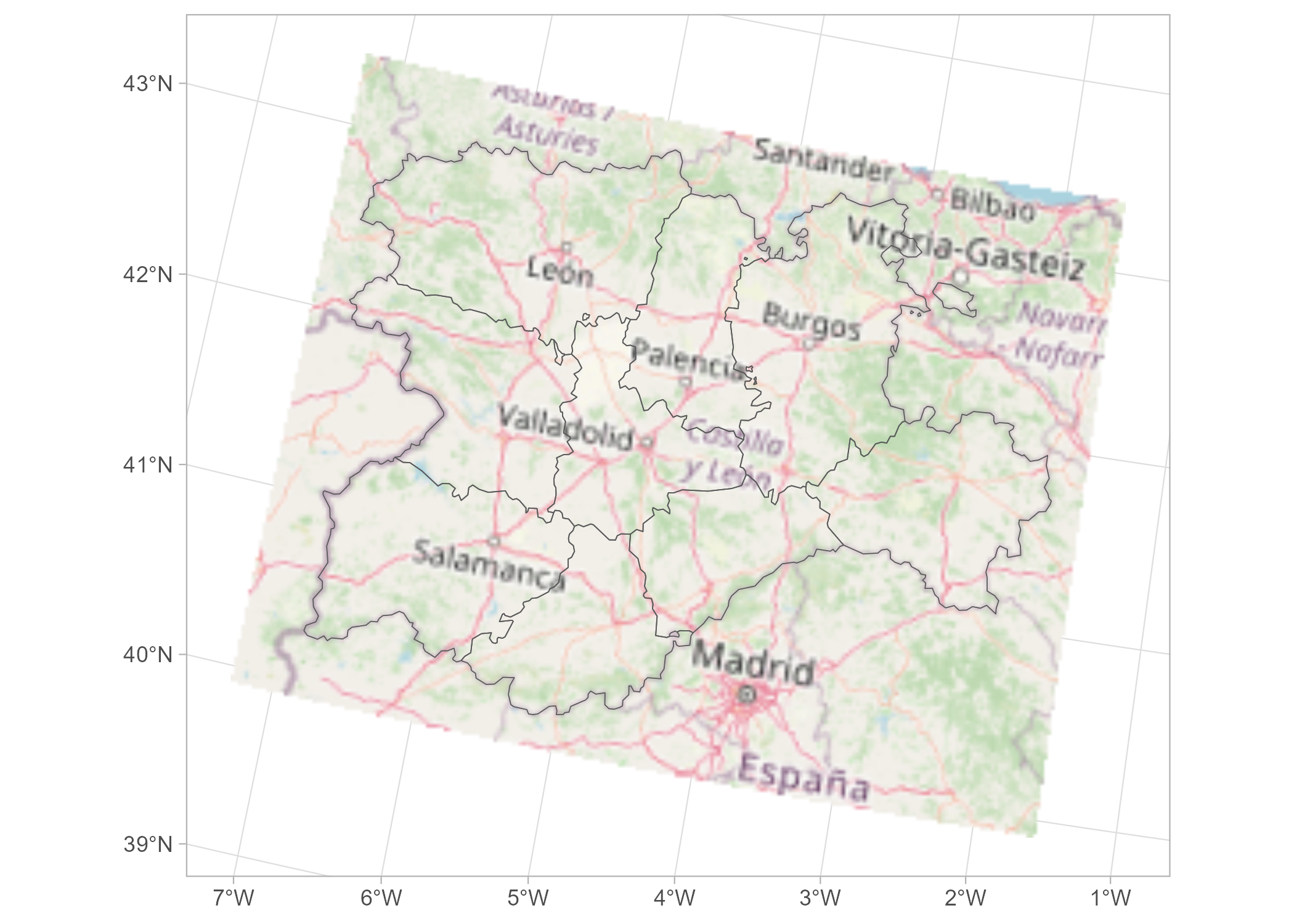``````
# Recognizes coord_sf()
plot +
# Change crs and datum (for relabeling graticules)
coord_sf(crs = 3857, datum = 3857)``````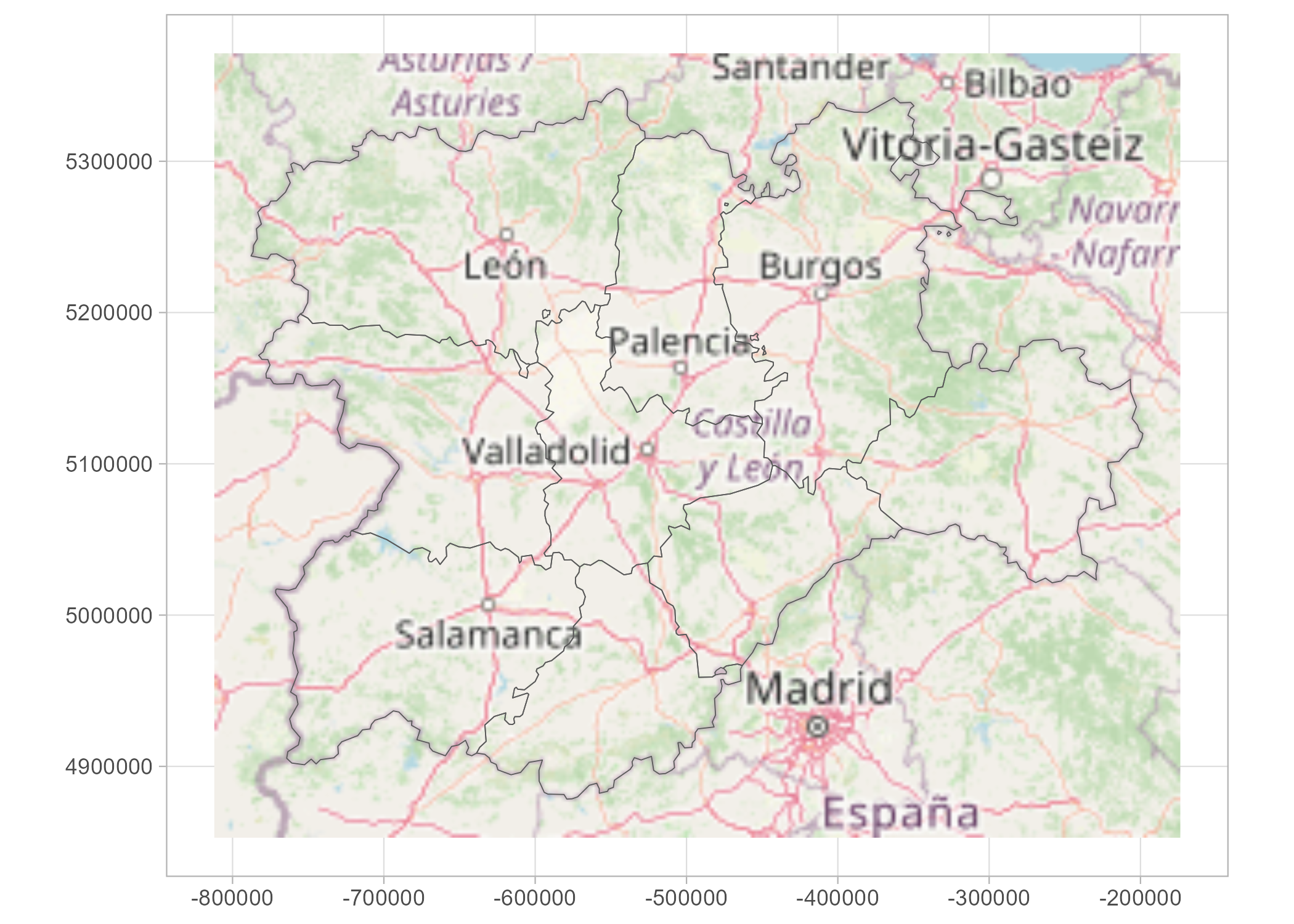{tidyterra} provides specific scales for plotting hypsometric maps with {ggplot2}:

``````asia <- rast(system.file("extdata/asia.tif", package = "tidyterra"))

terra::plot(asia)``````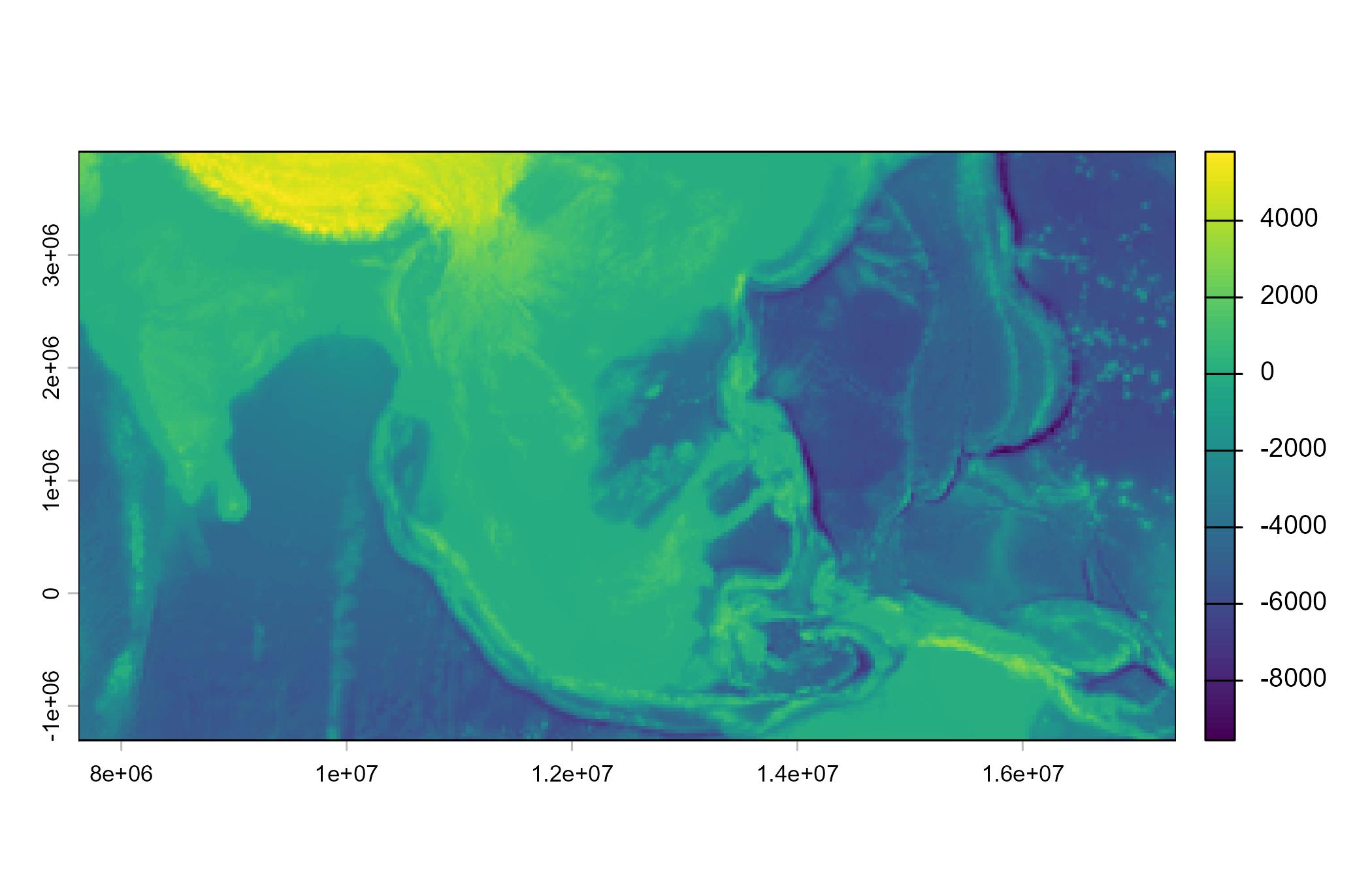``````
ggplot() +
geom_spatraster(data = asia) +
scale_fill_hypso_tint_c(
palette = "gmt_globe",
labels = scales::label_number(),
breaks = c(-10000, -5000, 0, 2500, 5000, 8000),
guide = guide_colorbar(
direction = "horizontal",
title.position = "top",
barwidth = 20
)
) +
labs(
fill = "elevation (m)",
title = "Hypsometric map of Asia"
) +
theme_minimal() +
theme(legend.position = "bottom")``````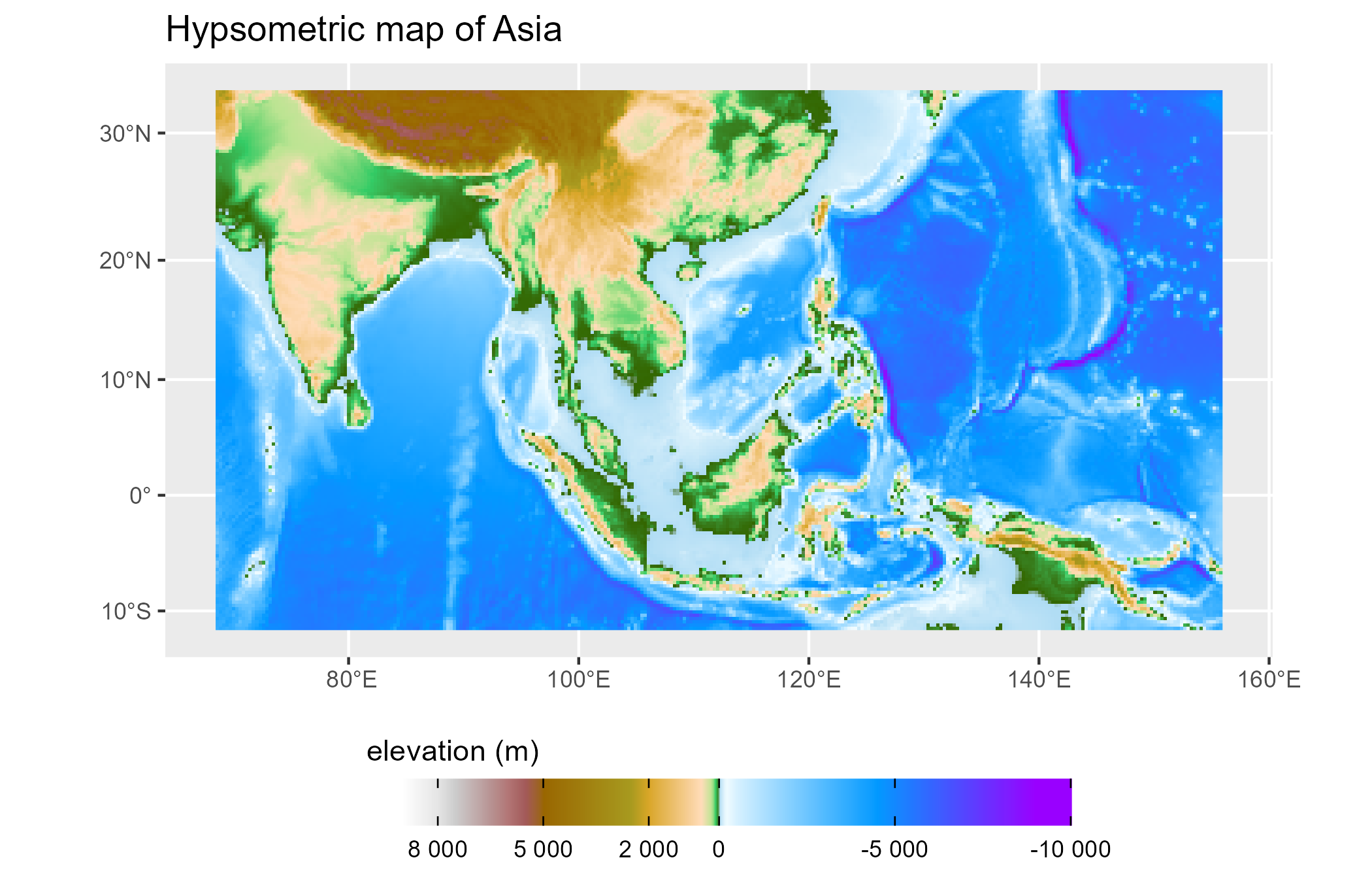### SpatVectors

This is a basic example which shows you how to manipulate and plot SpatVector objects:

``````vect(system.file("ex/lux.shp", package = "terra")) %>%
group_by(NAME_1) %>%
summarise(pop_dens = sum(POP) / sum(AREA)) %>%
glimpse() %>%
autoplot(aes(fill = pop_dens)) +
scale_fill_whitebox_c(palette = "pi_y_g")
#> Rows: 3
#> Columns: 2
#> \$ NAME_1   <chr> "Diekirch", "Grevenmacher", "Luxembourg"
#> \$ pop_dens <dbl> 80.83865, 134.90133, 485.34879``````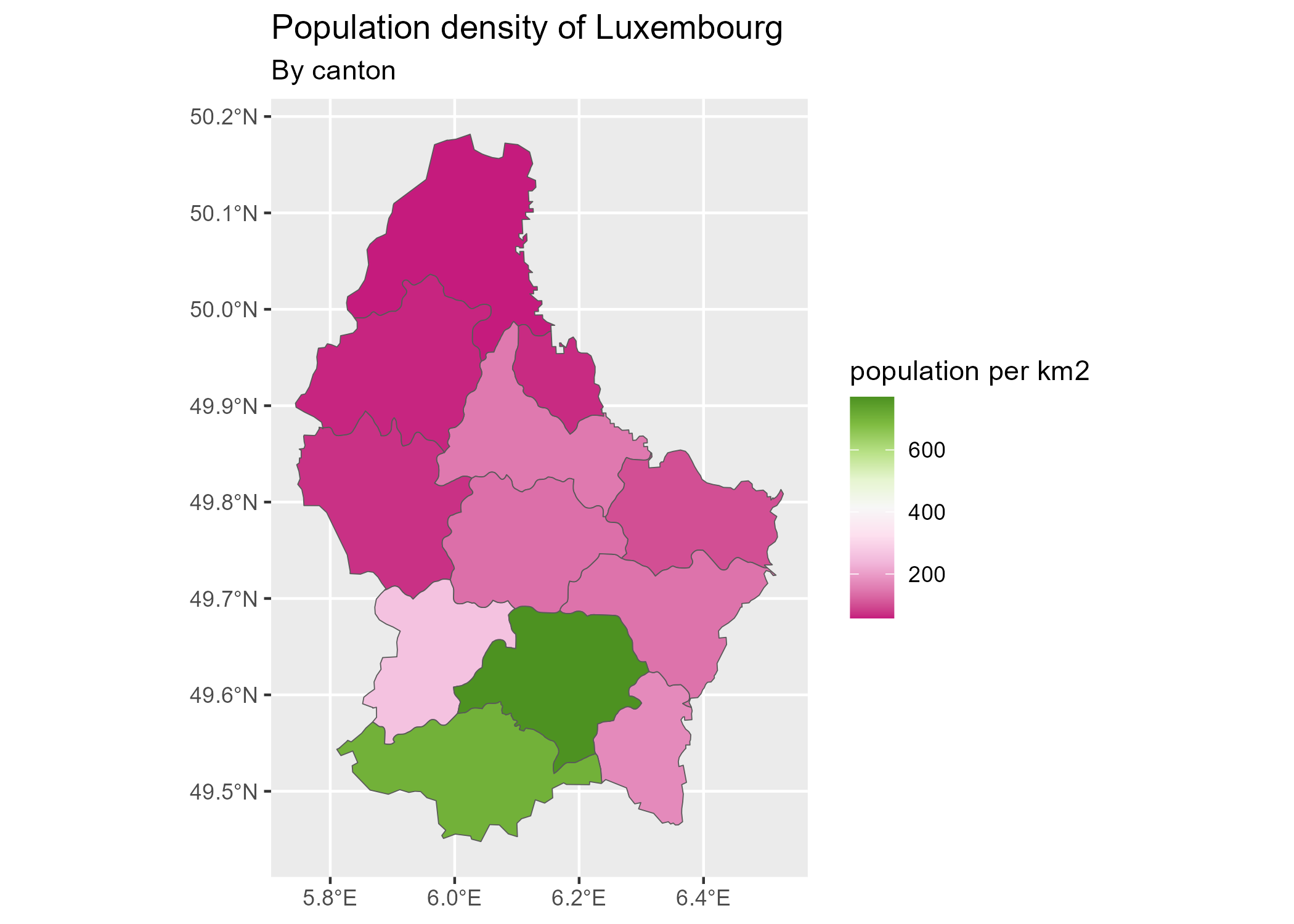{tidyterra} is currently on development mode. Please leave your feedback or open an issue on https://github.com/dieghernan/tidyterra/issues.

## Need help?

Check our FAQs or open a new issue!

## Citation

To cite ‘tidyterra’ in publications use:

Hernangomez D (2023). tidyterra: tidyverse Methods and ggplot2 Helpers for terra Objects. https://doi.org/10.5281/zenodo.6572471, https://dieghernan.github.io/tidyterra/

A BibTeX entry for LaTeX users is

``````@Manual{R-tidyterra,
title = {{tidyterra}: tidyverse Methods and ggplot2 Helpers for terra Objects},
doi = {10.5281/zenodo.6572471},
author = {Diego Hernangómez},
year = {2023},
version = {0.4.0},
url = {https://dieghernan.github.io/tidyterra/},
abstract = {Extension of the tidyverse for SpatRaster and SpatVector objects of the terra package. It includes also new geom_ functions that provide a convenient way of visualizing terra objects with ggplot2.},
}``````

## Acknowledgement

{tidyterra} ggplot2 geoms are based on {ggspatial} implementation, by Dewey Dunnington and ggspatial contributors.